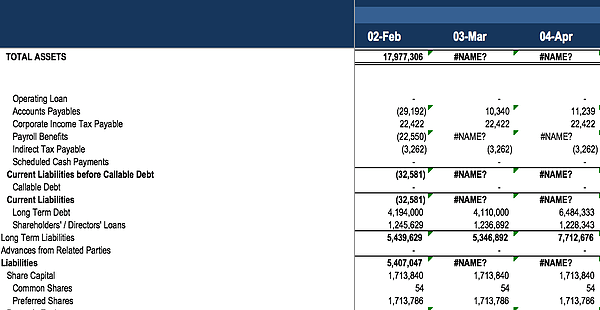# Stress Test – Financial Modeling

Finding the flaws in a financial model

## Why Stress Test a Financial Model?

The last key step in preparing a financial model is in stress testing it. The ability to stress test a financial model and find any flaws within it is a useful skill in improving the quality of a financial model. Stress testing also ensures no errors will occur in the future when the model has been handed off to the final user.

This final step is one that is often overlooked, even when stress testing a financial model prevents dissatisfied clients, managers, and executives.

### How to stress test in financial modeling?

One of the easiest methods of stress testing is to test the formula logic built into the calculations of the financial model. A simple sanity test will reveal whether the resulting values make sense. A more robust extension of this test is to fill the formula down or to the right into adjacent cells, and see whether the change properly flows through. Does the filled down formula result in appropriate values? If not, that may mean that there is an overlooked reference within the formula that must be adjusted. As shown in the image below, one of the lines in the statement is not referencing correctly. Stress testing reveals these errors.Because a financial model relies on the use of assumptions to calculate projected values, it becomes prudent to also stress test the formulas surrounding the assumptions. Test all probable and possible values of the assumptions and see if that crashes the formula. Decrease the assumption, increase it, flip its sign or make it zero, and see whether the formulas still calculate properly. If, in any case, the formula errors or becomes nonsensical, a further look into the formula logic may be required for that specific assumption.

If multiple users operate within the financial model, check the output values against their copy or version of the model. Are the assumptions similar and do the results match exactly? This is also a good time to ensure that proper formatting has carried over into the new iterations of the model.

### Error checking

Because of the structured nature of the core statements, it is not too difficult to ensure the data and calculations are correct. Below are just a few types of checks that can be placed within an Excel model to ensure that values are adding up correctly.

• Does the balance sheet add up? Do Assets minus Liabilities minus Equity equal zero?

• Does the change to retained earnings in the current period equal net income less dividends?

• Does the ending cash balance in the cash flow equal the cash balance in the balance sheet?

• Do ending values in the supporting schedules match their corresponding values in the core statements?

• One master error checks to determine whether all of the above are resulting correctly.

Placing these checks within a financial model allows the user to ensure calculations are being done correctly, and no formula logic has been made erroneously. The more checks in place, the more secure the financial model is from errors appearing.